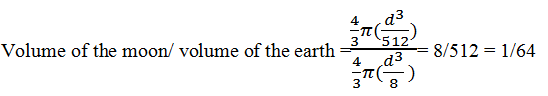Guru

# The diameter of the moon is approximately one-fourth of the diameter of the earth. What fraction of the volume of the earth is the volume of the moon? Q.4

• 0

How can i solve this tough question of class 9th ncert of Surface areas and Volumes of math of exercise 13.8   of question no.4. Give me the best and simple way for solving this question. The diameter of the moon is approximately one-fourth of the diameter of the earth. What fraction of the volume of the earth is the volume of the moon?

Share

1. Let the diameter of earth be “d”. Therefore, the radius of earth will be will be d/2

Diameter of moon will be d/4 and the radius of moon will be d/8

Find the volume of the moon :

Volume of the moon = (4/3) πr3 = (4/3) π (d/8)3 = 4/3π(d3/512)

Find the volume of the earth :

Volume of the earth = (4/3) πr3= (4/3) π (d/2)3 = 4/3π(d3/8)

Fraction of the volume of the earth is the volume of the moonAnswer: Volume of moon is of the 1/64 volume of earth.

• 0